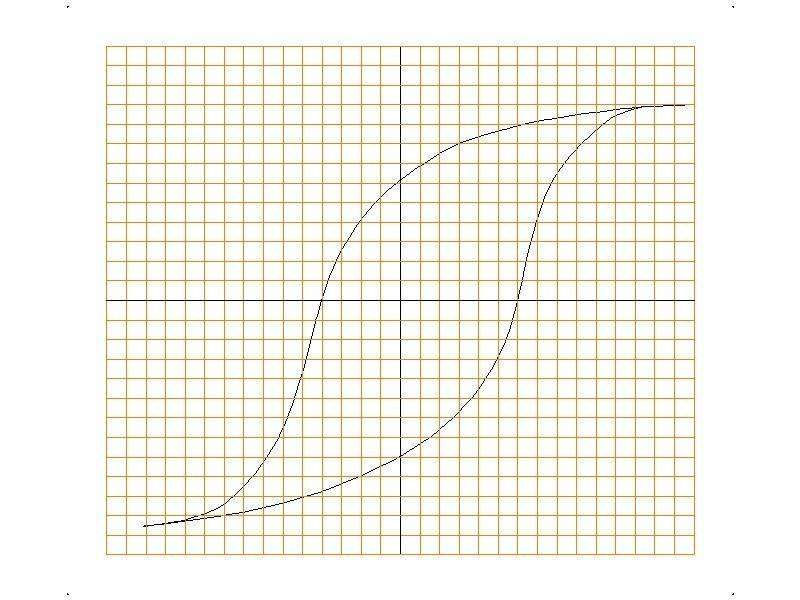# How to find Area of Hysteresis?

• I
• SDRFG
In summary: It's not just a quick howto - and it takes practise.In summary, you can use calculus to find the area under a curve, and then use numerical methods to find the best equation of the curve.
welcome to PF;
hint: use calculus.

•SDRFG
how to use calculus here i have never done .

SDRFG said:
how to use calculus here i have never done .
Then why do you need to know the area of the hysteresis loop?

•SDRFG
Then why do you need to know the area of the hysteresis loop?

I have studied calculus but no idea how too apply ?

Since you have studied calculus, you known how to use it to find the area under a curve.
That is pretty much the definition of "integration".

Perhaps if you ptovided an example?

•SDRFG
SDRFG said:
I have studied calculus but no idea how too apply ?

Does this ring any bells?

•SDRFG
If the hysteresis loop is plotted from test data then there will be no immediately available equations defining the shape of the up going and down going curves forming the loop .

To use calculus you need to find the equations of curves which are best fit to the plot curves . There are standard ways of doing this .

Alternatively you can use numerical methods . There are standard ways of doing this also .

•SDRFG
Numerical methods :

(1) Overlay the plot onto squared paper . Count the number of squares enclosed by the loop . Use a rule to decide when to include or exclude partial squares .

(2) Divide the plot into many slices with equally spaced horizontal or vertical lines . Estimate the area in each slice and sum .

(3) If you want to get more sophisticated look up : Trapezium Rule , Simpson's Rule and Gaussian Quadrature .

(Have a look at Gaussian Quadrature anyway for general interest - it is fascinating how a very small number of ordinate samples can give very accurate answers)

•SDRFG
When I started in the DO we sometimes used a Planimeter . Now we use facilities within our CAD software .

There are downloadable apps for working out areas enclosed by curves .

•SDRFG
OK, How to find equation of curve?

hello i understand planimeter

You could weigh the paper any calibrate it to the area. Fun experimental/hands on way to integrate without calculus, kids love it and you can talk about error.

The easy way :

http://uk.mathworks.com/products/curvefitting/

http://uk.mathworks.com/videos/curve-fitting-toolbox-overview-61198.html

(You can do the integration in Mathworks as well if you want to)

The moderately easy way :

Choose simple functions which give the right sort of curve shapes to fit your plot and scale them to best fit . Sometimes easier to break plot curve into segments and use different functions for each segment .

Commonly used functions : linear , polynomial , exponential and sine/cosine .

Slightly more formal way :

https://en.wikipedia.org/wiki/Curve_fitting#Fitting_lines_and_polynomial_functions_to_data_points

•SDRFG
•SDRFG
hello,

How to drawn second degree polynomial, like this?

SDRFG said:
How to drawn second degree polynomial, like this?
SDRFG, can you attach a neat plot of your hysteresis loop, with the measured points clearly shown?

Do you intend using a software package to find a polynomial of best fit? (I expect wolfram alpha would do this for you.)

•SDRFG
i want to learn by hand in paper

SDRFG said:
i want to learn by hand in paper

•SDRFG
I don't have any plot it was in college i left it over 3 year completing degree. they dnt guide properly may be lack of information.

SDRFG said:
I don't have any plot it was in college i left it over 3 year completing degree. they dnt guide properly may be lack of information.
That's okay. It's obviously a matter that piqued your interest at the time, and you kept it in mind should the opportunity later present itself to pursue it further.

Here's your opportunity. What would you like to do in this thread at this point?

•SDRFG
Here's your opportunity. What would you like to do in this thread at this point?
At class i was not aware of it, but while teaching for few days on it it attract me.
and without this basic i can't move further it involve practical math.

So what would you like to be the next step in this thread today?

You are happy with the answers you have received so far? Can we assist you further?

•SDRFG
yes, i get my answer you can assist further.

If you have a set of data points, you can fit a polynomial to that set by using "least squares regression".
Google for: "least squares curve fitting lecture", and see if you can find a lesson that suits you. ie.
http://web.iitd.ac.in/~pmvs/courses/mel705/curvefitting.pdf
It's not just a quick howto - and it takes practise.

If you have ever done linear regression, it is like that only for curves.

It is usually quite time consuming so most people will use a computer to do this - usually matlab, octave, or mathatica.
https://www.gnu.org/software/octave/

Apart from that: how can we assist you further?

•SDRFGCAD scan of the curves overlaid on a CAD generated grid . You can now try out some of the suggested methods for finding enclosed area if you want to .

Last edited:
•SDRFG